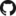# PredcompsHome

PDF Manual

Per Unit Input (original APC)

Impact (in units of output)

## Examples

Examples Overview

A fake logistic regression example predicting wine sales

A linear model with interactions

Loan defaults example

## More

As compared with Gelman & Pardoe 2007

Pairs & Weights

## Examples Overview

### Simulated Examples

The simulated examples are constructed to demonstrate properties of predictive comparisons in artificial simulations that we can perfectly understand (because we created them).

• Logistic regression for wine prices: This is a logistic regression model run in different simulated data situations. Changing the relationship between the inputs (and nothing else) leads to differences in the predictive comparisons, despite the logistic regression model remaining the same.

• Linear model with interactions: This is a linear model with 9 features and 8 interaction terms (an interaction between $$v$$ and each of $$u_1 \ldots u_8$$) which are all the same. The way the $$u_i$$'s are related to $$v$$ in the distribution of the inputs gives rise to differences in the APC, despite each $$u_i$$ playing the same role in the model.

### Real Examples

Simulated examples are good for gaining understanding of how the package works and what it's doing, but they don't help us understand how it is useful in the real world. These are examples with real-world data sets:

• Loan Default Model: In this example, applying predictive comparisons to a random forest helps understand the drivers of a credit risk model.